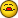# Use & with two formulas

L

#### lhkittle

With these two formulas, I can make them work okay if I select four cells and enter a formula and array enter. Select four more cells and do the same with the second.

Where A2 holds 4,6

=VLOOKUP(--LEFT(A2,1),A49,{2,3,15,16},0)
=VLOOKUP(--RIGHT(A2,1),A49,{2,3,15,16},0)

I thought I could select eight cells and enter the formulas as:

=VLOOKUP(--LEFT(A2,1),A49,{2,3,15,16},0)&VLOOKUP(--RIGHT(A2,1),A49,{2,3,15,16},0)

When I use eight cells, the first formula seems to return its stuff and I get #N/A's for the second four cells.

I've use & in the past and it worked, but here I am drawing a blank.

Thanks,
Howard

C

#### Claus Busch

Hi Howard,

Am Fri, 12 Jul 2013 11:31:09 -0700 (PDT) schrieb (e-mail address removed):
=VLOOKUP(--LEFT(A2,1),A49,{2,3,15,16},0)
=VLOOKUP(--RIGHT(A2,1),A49,{2,3,15,16},0)

what do your want to do?
Select 4 cells and try:
=VLOOKUP(INT(A2),A49,{2,3,15,16},0)&"/"&VLOOKUP(--RIGHT(A2,1),A49,{2,3,15,16},0)

Regards
Claus B.

L

#### lhkittle

Hi Howard,

Am Fri, 12 Jul 2013 11:31:09 -0700 (PDT) schrieb (e-mail address removed):

what do your want to do?

Select 4 cells and try:

=VLOOKUP(INT(A2),A49,{2,3,15,16},0)&"/"&VLOOKUP(--RIGHT(A2,1),A49,{2,3,15,16},0)

Regards

Claus B.

Whoa!! That result was unexpected, I had it in my mind that the eight return values would be in eight separate cells. In four cells is fine along with the / between.

And now that I reflect on it this is pretty much what was returned in my claim of having used & with two formula similar to this before.

Thanks, Claus.

Regards,
Howard